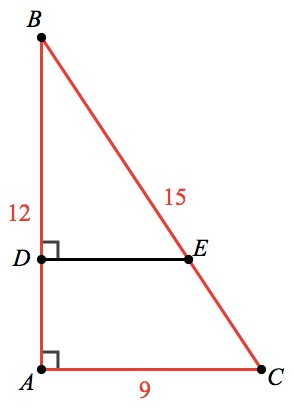Test 7 Section 4 #36

Two important concepts in this question, closely related: trigonometry and Pythagorean triples.

First, the trig. The fact thatmeans that the short legs of both triangles in the question arethe lengths of the longer legs.

Now the Pythagorean triples: 3-4-5 is the most important Pythagorean triple to know! It’s called a Pythagorean triple because it’s a case where three integers work in the Pythagorean theorem:. If you know the legs of a right triangle are in a 3-4 ratio, then you know that you’ve got a 3-4-5 triangle (or one of its bigger cousins)!

The question tells you that BC = 15. That’s the hypotenuse of the longer side, so your larger triangle is a 9-12-15 (AKA a 3-4-5 times 3). So AC = 9 and AB = 12.If DA = 4, that means DB = 12 – 4 = 8. This means the smaller of the triangles you’re dealing with is a 6-8-10 (AKA a 3-4-5 times 2). You’re asked for the value of DE, which must therefore be 6.